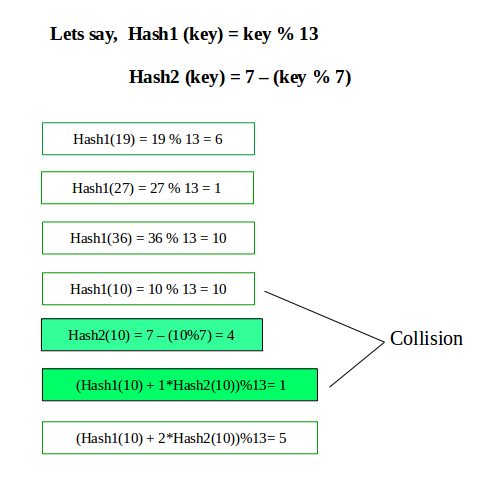# Double Hashing

Double hashing is a collision resolving technique in Open Addressed Hash tables. Double hashing uses the idea of applying a second hash function to key when a collision occurs.

• The advantage of Double hashing is that it is one of the best form of probing, producing a uniform distribution of records throughout a hash table.
• This technique does not yield any clusters.
• It is one of effective method for resolving collisions.

Double hashing can be done using :
(hash1(key) + i * hash2(key)) % TABLE_SIZE
Here hash1() and hash2() are hash functions and TABLE_SIZE
is size of hash table.
(We repeat by increasing i when collision occurs)

First hash function is typically hash1(key) = key % TABLE_SIZE
A popular second hash function is : hash2(key) = PRIME – (key % PRIME) where PRIME is a prime smaller than the TABLE_SIZE.
A good second Hash function is:

• It must never evaluate to zero
• Must make sure that all cells can be probed## CPP

 `/*` `** Handling of collision via open addressing` `** Method for Probing: Double Hashing` `*/`   `#include ` `#include ` `#include ` `using` `namespace` `std;` `#define MAX_SIZE 10000001ll`   `class` `doubleHash {`   `    ``int` `TABLE_SIZE, keysPresent, PRIME;` `    ``vector<``int``> hashTable;` `    ``bitset isPrime;`   `    ``/* Function to set sieve of Eratosthenes. */` `    ``void` `__setSieve(){` `        ``isPrime = isPrime = 1;` `        ``for``(``long` `long` `i = 2; i*i <= MAX_SIZE; i++)` `            ``if``(isPrime[i] == 0)` `                ``for``(``long` `long` `j = i*i; j <= MAX_SIZE; j += i)` `                    ``isPrime[j] = 1;`   `    ``}`   `    ``int` `inline` `hash1(``int` `value){` `        ``return` `value%TABLE_SIZE;` `    ``}` `    `  `    ``int` `inline` `hash2(``int` `value){       ` `        ``return` `PRIME - (value%PRIME);` `    ``}`   `    ``bool` `inline` `isFull(){` `        ``return` `(TABLE_SIZE == keysPresent);` `    ``}`   `    ``public``: `   `    ``doubleHash(``int` `n){` `        ``__setSieve();` `        ``TABLE_SIZE = n;`   `        ``/* Find the largest prime number smaller than hash table's size. */` `        ``PRIME = TABLE_SIZE - 1;` `        ``while``(isPrime[PRIME] == 1)` `            ``PRIME--;`   `        ``keysPresent = 0;`   `        ``/* Fill the hash table with -1 (empty entries). */` `        ``for``(``int` `i = 0; i < TABLE_SIZE; i++)` `            ``hashTable.push_back(-1); ` `    ``}`   `    ``void` `__printPrime(``long` `long` `n){` `        ``for``(``long` `long` `i = 0; i <= n; i++)` `            ``if``(isPrime[i] == 0)` `                ``cout<

Output

```Status of hash table after initial insertions : -1, 66, -1, -1, -1, -1, 123, -1, -1, 87, -1, 115, 12,

Search operation after insertion :
12 present
115 present
Status of hash table after deleting elements : -1, -2, -1, -1, -1, -1, -2, -1, -1, -2, -1, 115, 12, ```

My Personal Notes arrow_drop_up
Recommended Articles
Page :